Visualizing Solutions to the Beam Equation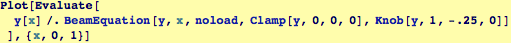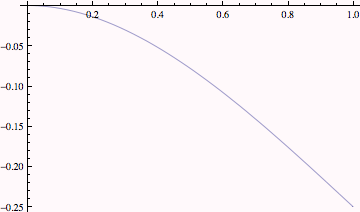BeamViz: Visualization Function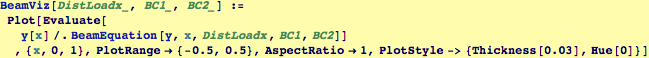Unit load pushing up, a clamp at the left and a free left end: This is a first-order model for the deflection of an airplane wing.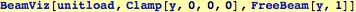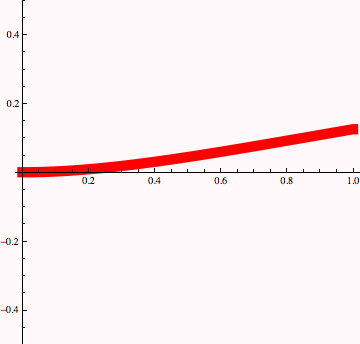Fixed Knobs that rotate freely at both ends, load: Approximates the defllection of a sieve loaded by  shearing flow.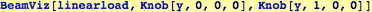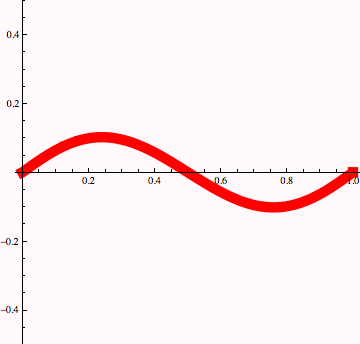A box of width 1/8 sitting at x=3/4 for a double cantilever beam.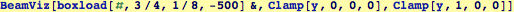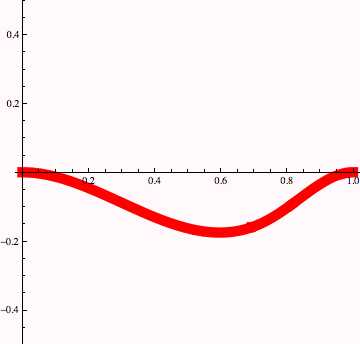A point load at the center of a beam clamped at the left and sitting on a pinned point at the right.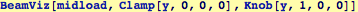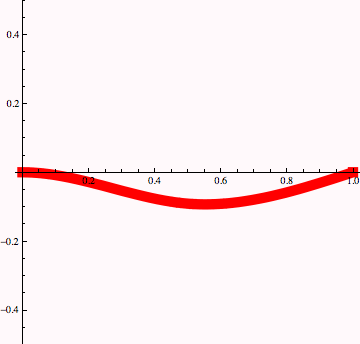A load applied to the center with balancing loads applied at the ends: Three Point Loading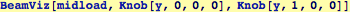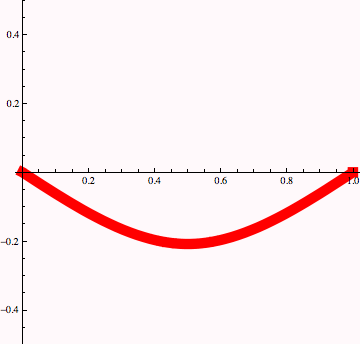Created by Wolfram Mathematica 6.0  (16 November 2007)